# 4. The Visu Models

## 4. The Visu Models

We present in this section the two models of the VISU system, which allows both to annotate videos with high level semantic descriptions and to query these descriptions for generating video summaries. Thus, the VISU System relies on two models: an annotation model which uses strata and conceptual graphs for representing annotations, and a query model, based on SQL, for describing the expected summary. The query processing exploits an efficient graph matching algorithm for extracting the frames or sequences of frames which answer the query before to generate the summary according to some relevance criteria. We describe here the VISU models and the process of summary generation.

### 4.1. The Annotation-Based Structure Model

In this model, objects, events and actions occurring in a video are representation units linked to strata similar to those presented in section 2. Moreover, we organize these representation units in Conceptual Graphs wherein nodes correspond to concepts, events or/and actions which are linked together in complex structures. A video or a segment of video can be annotated using several strata. Each stratum is associated with a set of chronologically ordered segments of video. Then, as shown in Figure 11.1, two strata involved in a video may share some common segments of video, meaning that the object, event or action they respectively represent both appear simultaneously in those segments. Provided that, for each segment of video where it occurs, a stratum is associated with the starting time and the ending time of this segment, the overlapping segments can be computed as the intersection of the two sets of segments.Figure 11.1: An example of annotation-based structure for a video. A video of 300 frames is here annotated using 2 initial strata, namely Strata1 and Strata2. The video segments between frames 87 and 165 are related only to the description of stratum 2; the frame intervals [166, 177] and [193,229] are described by a generated strata Strata3, corresponding to a conjunction of the annotations of the strata 1 and 2, where the frame intervals [178,192] and [230,246] are described only by the stratum 1.

Annotations associated with strata can be seen made of elementary descriptions (representation units) or more complex descriptions organized in Conceptual Graphs . The Conceptual Graph formalism is based on a graphical representation of knowledge. Conceptual Graphs can express complex representations, and are able to be used efficiently for retrieval purposes .

More formally, Conceptual graphs are bipartite oriented graphs, composed of two kinds of nodes: concepts and relations.

• A Concept, noted "[T: r]" in an alphanumeric way and T: r in a graphical way, is composed of a concept type T and a referent r. Concept types are organized in a lattice that represents a generic/specific partial order. For the concept to be correct syntactically, the referent has to conform to the concept type, according to the predefined conformance relationship. Figure 11.2 shows a simple lattice that describes the concept types Person, Woman and Man; Tc and C represent respectively the most generic and the most specific concepts of the lattice. A referent r may be individual (i.e., representing one uniquely identified instance of the concept type), or generic (i.e., representing any instance of the concept type, and noted by a star: "*").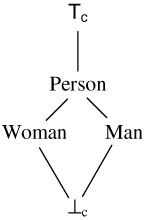Figure 11.2: An example of concept types lattice.

• A relation R is noted "(R)" in a alphanumeric way and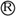in a graphical way. Relations are also represented in a lattice expressing a generic/specific partial order.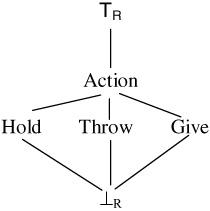Figure 11.3: An example of relations lattice

We call arch a triplet (concept, relation, concept) that links three nodes including one relation and two concepts in a conceptual graph. In the following, we refer to a triplet "conceptrelationconcept" as an arch.

Conceptual graphs can be used to represent complex descriptions. For instance, the Conceptual Graph G1, noted [Man: #John](talk_to)[Woman: #Mary] can describe the semantic content of a frame or segment of frames. In this description, [Man: #John] represents the occurrence of a man, John, [Woman: #Mary] represents the occurrence of a woman, Mary, and the graph (which here, reduces to a simple arch) expresses the fact that John is talking to Mary. Figure 11.4 presents the graphical representation of this simple graph. It can be noticed that the expressive power of Conceptual Graphs is able to represent hierarchies of concepts and relations between objects as proposed by the MPEG-7 committee.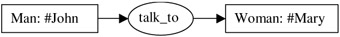Figure 11.4: An example of conceptual graph.

Syntactically correct Conceptual Graphs are called Canonical Graphs. They are built using a set of basic graphs that constitutes the Canonical Base, from the following construction operators:

• joint: this operator joins graphs that contain an identical (same concept type and referent) concept,

• the restriction operator constrains a concept by replacing a generic referent by a specific one,

• the simplification operator removes duplicate relations that may occur after a joint operation, for instance,

• the copy operator copies a graph.

As shown by Sowa, the advantage of using conceptual graphs is that a translation, noted φ, exists between these graphs and the first order logic, providing conceptual graphs with a sound semantics. We exploit this property to ensure the validity of the summary generation process. This process is based on the material implication of first order logic (see ).

According to the conceptual graphs formalism described above, the joint operator can be used to achieve the fusion of graphs when possible. Thus, the resulting joint graph G of two graphs Gi and Gj, performed on one concept Cik of Gi and one concept Cjl of Gj, where Cik is identical to Cjl, is such that:

• every concept of Gi is in G

• every concept of Gj except Cjl is in G

• every arch of Gj containing Cjl is transformed in G in an arch containing Cik

• every arch of Gi is in G

• every arch of Gj that do not contain Cjl, is in G

As described above, an annotation of a strata may correspond to a single graph description, or to a conjunction of graphs under the form of one graph or a non-singleton set of graphs. More precisely, consider for instance two annotations s1 and s2 described respectively by the graphs gl, "[Man: John]->(talk_to)->[Woman: Mary]," and g2, "[Man: John]->(playing)->[Piano: piano1]." If the video segments corresponding to the related strata intersect, then the graph describing the generated stratum from the strata related to g1 and g2 is the graph g3, i.e. the graph that join g1 and g2 on their common concept [Man: John], as presented in Figure 11.5. The graph g3 is the best representation for the conjunction of the graphs g1 and g2, since in g3 we explicitly express the fact that the same person (John) is playing and talking at the same time. If we consider now two descriptions g3 "[Man: John]->(talk_to)->[Woman: Mary]" and g4 "[Man: Harry]->(playing)->[Piano: piano1]," then the description that corresponds to the generated strata cannot be the result of a joint operation because the two graphs g3 and g4 do not have a common concept, and then the annotation of the intersection is the set { g3, g4}. Generated strata are necessary to avoid mismatches between query expressions and initial strata graph descriptions.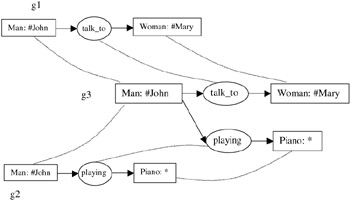Figure 11.5: Joint of two graphs (g1 and g2) on a concept [Man— #John].

Figure 11.5 describes the joint of two graphs but it should be noticed that in our annotation-based structure, we try to joint all the graphs that correspond to generated strata. Such a task is simply achieved through an iterative process that first finds the identical concepts of strata, and then generates joint graphs when possible. If no joint is possible for some graphs, then these graphs are kept such as they are in the set of graphs describing the generated stratum.

Our objective is to exploit the complex description of Conceptual Graphs involving strata in order to generate meaningful summaries. This goal requires associating a measure of the relative importance of the graph elements: the concepts and the arches. Concepts are important because they express the basic elements present in the description. But arches are important too because they represent relationships between elements. The solution adopted here is based on the weighting scheme of the well known tf*idf (term frequency * inverse document frequency) product used for 30 years in the text-based information retrieval domain . The term frequency is related to the importance of a term in a document, while the inverse document frequency is related to the term's power to distinguish documents in a corpus. Historically, these tf and idf values were defined for words, but here, we use them to evaluate the relevance of concepts and arches in graphs. We describe below how these values are computed, respectively, for a concept and an arch, in a Conceptual Graph:

• The term frequency tf of a concept C in a conceptual graph description Gsj is defined as the number of concepts in Gsj that are specific concepts of C. A concept X, [typeX : referentX] is a specific of Y, [typeY : referentY], if typeX is a specific of typeY according to the lattice of concept type (i.e., typeX is a sub-type of typeY) and referentX=referentY (they represent the same individual) or referentX is an individual referent and referentY is the generic referent '*'. In the graph "[Man: #John]->(talk_to)->[Man: *]," representing the fact that John is talking to an unidentified man, tf([Man: #John]) is equal to 1, and tf([Man: *]) is equal to 2 because both [Man: #John] and [Man: *] are specific of [Man: *].

• The inverse document frequency idf of a concept C is based on the relative duration of the video parts that are described by a specific of C, using a formula inspired from : idf(c)=log(1+D/d(c)) with D the duration of the video and d(C) the duration of the occurrence of C or a specific of C. For instance, if John appears on 10% of the video idf([Man: #John])=1.04, and if a Man occurs 60% of the video, idf([Man: *])=0.43.

• For an arch A, the principle is similar to concepts: the term frequency tf(A) is based on the number of different arches that are specific of A in a considered graph. An arch (C1x, Rx, C2x) is a specific of an arch (C1y, Ry, C2y) if and only if the concept C1x is a specific of C1y, and if the concept C2x is a specific of the concept C2y, and if the relation Rx is a specific of the relation Ry according to the relation lattice (the record-type associated to Rx is a sub-record-type of the record-type associated to Ry).

• The idf of an arch A is defined similarly to concepts, and is based on the relative duration of the video parts that are described by specific arches of A.

### 4.2. Cinematographic Structure Model

The cinematographic structure Model is dedicated to represent the organization of the video according to what we presented in part 2.3. This structure is a tree that reflects the compositional aspect of the video; the node corresponds to a structural level and a frame number interval. The inclusion of parts is based on the interval inclusion.

As explained previously, one video is structured into scenes, shots and frames. We choose to limit the structure to shots and frames, because according to the state of the art shot boundaries can be extracted with an accuracy greater than 95% (at least for cuts, as described in ), while automatic scene detection is still not effective enough for our needs.

### 4.3. Query Model

We use a query model in order to describe the expected content of the adaptive summary and the expected duration of the summary through the formulation of a query. For instance, a query Q1 that expresses the fact that we look for video parts where John talks to somebody can be written: "[Man: #John](talk_to)[Human: *]." It is obvious that the graph G1 presented in section 4.1.1 and noted [Man: #John](talk_to)[Woman: #Mary] is an answer for the query Q1. In the context of VISU, this means that a frame or segment of frames (assuming that duration constraints are satisfied) described by the graph G1 should be present in a summary described by means of the query Q1.

In the query model of VISU, we also allow users to assign weights to the different parts of the query. These weights reflect the relative importance of different contents of the videos. A syntax of a query is given in Figure 11.6, using the usual Backus-Naur semantics for the symbols "[", "]", "{", "}" and "*".` SUMMARY FROM video WHERE graph [WITH PRIORITY {HIGH|MEDIUM|LOW}]       [ {AND|OR|NOT} graph [WITH PRIORITY {HIGH|MEDIUM|LOW}] ]* DURATION integer {s|m} `Figure 11.6: Query syntax.

In Figure 11.6, video denotes the initial video that is to be summarized. Graph is represented as a set of arches in an alphanumerical linear form. An arch is represented by "[Type1: referent1 | id1]->(relation)->[Type2: referent2 | id2]," where the concept identifiers id1 and id2 define uniquely each concept in a way to represent concepts that occur in more than one arch. The concept identifiers are used because a linear form is not able without such identifiers to fully represent graphs, particularly when considering generic referents. For instance, a graph representing that a man, John, is talking to a unidentified woman, and at the same time John is smiling to another unidentified woman, is represented as: "{[Man: John | 0]->(talk_to)->[Woman: *| 1], [Man: John | 0]->(smile)->[Woman: *|2]}." Without such identifiers, it would not be possible to distinguish between the fact that John smiles to the same woman or to another woman, integer after the keyword DURATION corresponds to the expected time of the summary with the unit s for seconds and m for minutes.

To illustrate, let us consider that we want to obtain a summary:

• taken from the video named "Vid001"

• showing a man, John, is talking to a woman, Mary, and at the same time John is at the right of Mary, with a high importance

• or showing snow falling on houses

• and having a duration of 20 seconds.

The query which corresponds to the expected generation can be formulated as follows:

` SUMMARY FROM Vid001 WHERE {[Man: John|0]->(talk_to)->[Woman: Mary| 1],         [Man: John|0]->(right_of)->[Woman: Mary| 1]}WITH PRIORITY HIGH   OR {{[SNOW:*|0]->(falling)->[BUILDING:*|1]} WITH PRIORITY MEDIUM DURATION 20s `

### 4.4. Summary Generation with VISU

The query processing relies on two phases. One is dedicated to the video content and the other is dedicated to the duration of the resulting summary. We describe each of these phases in the following.

#### 4.4.1. Information Retrieval theoretical background

To evaluate the relevance value between a query Qi and a document stratum Dj, we consider the use of the logical model of information retrieval . This meta-model stipulates, in the Logical Uncertainty Principle, that the evaluation of the relevance of a document (represented by the sentence y) to a query (represented by a sentence x) is based on "the minimal extend" to be added to the data set in order to assess the certainty of the implication xy. In our case the xy is related to the existing knowledge in the Conceptual Graph Canon (composed of the canonical base, the concept type hierarchy, the relation hierarchy and the conformance relationship), and the "" symbol is the material implication of first order logic. In our process, once the information of an annotation implies an annotation the relevance value of a stratum Sj according to a query Qi can then be computed as we explain in the following.

#### 4.4.2. Matching of graphs

The conceptual graph formalism allows to search in graphs using the project operator . The project operator is equivalent to the material implication according to the semantics given to conceptual graphs. The project operator, a sub-graph search, takes into account the hierarchy of concepts types and of relations. The projection of a query graph Gqi, noted πGsj(Gqi), into a conceptual graph Gsj, only concludes on the existence of a sub-graph in the description that is specific to the query graph. In the work of , it has been shown that the projection on graphs can be implemented very efficiently in term of search algorithm complexity.

We quantify the matching between a content query graph Gq and an annotation graph Gs by combining concepts matching and arches matching, inspired by  and :

 (1)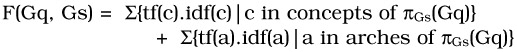In formula (1), the tf and idf concepts and arches are defined according to section 4.1.1.

Since we defined that an annotation may be one graph or a set of conceptual graphs, we now have to express how to match a query graph and a set of graphs corresponding to an annotation. Consider an annotation composed of a set S of graphs Gs, 1kN. The match M of the query graph Gq and S is:

M(Gq,S) = maxGSS (F(Gq,Gs))

#### 4.4.3. Query expression evaluation

We describe here how the priorities in the sub-query expression "{graph} WITH PRIORITY P," P being "HIGH," "MEDIUM" or "LOW" are taken into account in order to reflect the importance of the sub-expression for the summary generation. The notion of priority reflects the importance of the different sub-parts of the query content, and was defined in the MULTOS system  or in the OFFICER system . A query sub-expression is then evaluated for each graph of each annotation of the annotation-based structure, using a simple multiplication rule: if the matching value obtained for a node is v, then the matching value for the query sub-expression is: v p, with p equal to 0.3 for "LOW," 0.6 for "MEDIUM" and 1.0 for "HIGH."

Complex query expressions composed by Boolean expressions of elementary sub-query expressions are evaluated for strata annotations using Lukasiewicz-like definitions for n-valued logics:

• an expression composed of "A AND B" is evaluated as the minimum of the matching for each sub-queries A and B,

• an expression composed of "A OR B" is evaluated as the maximum of the matching values of each sub-query A and B,

• an expression composed of "NOT B" is evaluated as the negative of the matching value for B.

Then, we are able to give a global matching value for each of the annotations of the annotation-based structure.

The duration part of the query is used to constrain the result to a given duration. Three cases might arise:

• The duration of all the relevant video parts is larger than the duration expected. Then we avoid presenting the video parts that are less relevant, i.e., the video parts that have the lower matching values. We notice, however, that this is only processed for video parts that match with a positive matching value for the summary generation criteria.

• The duration of all the relevant video parts is equal to the duration expected. Then the result is generated with all the relevant video parts.

• The duration of the relevant video parts is smaller than the duration expected. Then the result is generated using all the relevant parts obtained and using the cinematographical structure of the video: consider that the query asks for x seconds and that the duration of the relevant semantic parts is y seconds (we have then y < x by hypothesis). The remaining video to add to the relevant parts must be x-y seconds long. We include in the result continuous excerpts of (x-y)/n seconds for each of the n shots that do not intersect with any relevant video parts. The excerpts correspond to the shot parts having the more motion activity in each shot, consistently with the work of  on signal-based summarization.

In any case, we force the result generated to be monotonic according to the frame sequence: for each frame fri and frj in the generated result corresponding respectively to the frames fok and fo1 in the original video, if the frame fok is before (resp. after) the frame fo1 in the original video, then the frame fri is before (resp. after) the frame frj in the generated result.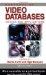Handbook of Video Databases: Design and Applications (Internet and Communications)
ISBN: 084937006X
EAN: 2147483647
Year: 2003
Pages: 393

flylib.com © 2008-2017.
If you may any questions please contact us: flylib@qtcs.net